Select Page

# CBSE MCQ in English Solutions for Maths 12 Science Differential Equations

CBSE MCQ in English Solutions for Maths 12 Science Differential Equations to enable students to get Solutions in a narrative video format for the specific question.

Expert Teacher provides CBSE MCQ Solutions for Maths 12 Science Differential Equations through Video Solutions in English language. This video solution will be useful for students to understand how to write an answer in exam in order to score more marks. This teacher uses a narrative style for a question from Differential Equations not only to explain the proper method of answering question, but deriving right answer too.

Please find the question below and view the Solution in a narrative video format.

Question:

Solution Video in English:

You can select video Solutions from other languages also. Please check Solutions in ( Hindi )

## Similar Questions from CBSE, 12th Science, Maths, Differential Equations

Question 1 : Determine degree of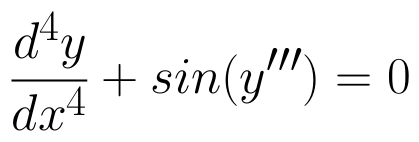(View Answer Video)

Question 2 : Solve the differential equation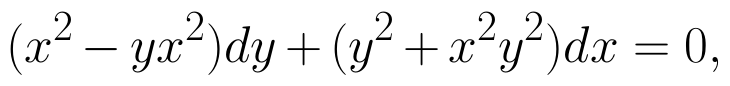given that y = 1 when x = 1. (View Answer Video)

Question 3 : Form the differential equation of the family of circles touching the x-axis at origin .  (View Answer Video)

Question 4 : Show that the solution of differential equation :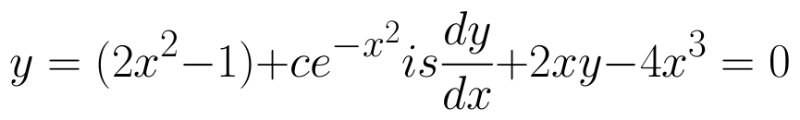(View Answer Video)

Question 5 : Find the particular solution of the differential equation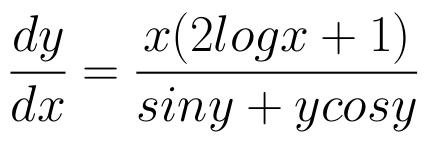given that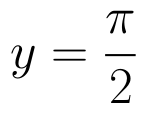where x = 1. (View Answer Video)

### Matrices

Question 1 : Let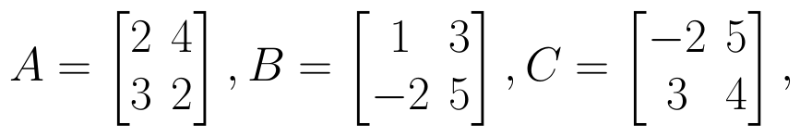Find A - B. (View Answer Video)

Question 2 : Find the value of x,  from the equation: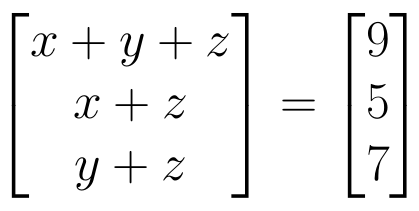. (View Answer Video)

Question 3 : If a matrix has 24 elements, what are the possible orders it can have? (View Answer Video)

Question 4 : Find the value of c from the equation: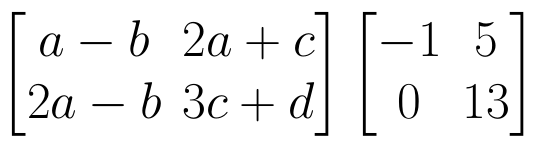(View Answer Video)

Question 5 : If,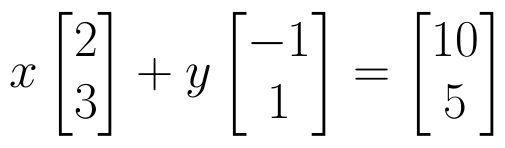find the value of y.  (View Answer Video)

### Relations and Functions

Question 1 : Let * be the binary operation on N given by a * b = LCM of a and b. Find 5 * 7. (View Answer Video)

Question 2 : A functiondefined as f(x) = 5x is, (View Answer Video)

Question 3 : Considergiven by f(x) = 4x + 3. Find the inverse of f. (View Answer Video)

Question 4 : Let * be the binary operation on N given by a * b = LCM of a and b. Find 20 * 16. (View Answer Video)

Question 5 :  Let R be the relation on the set {1, 2, 3, 4} given by R = {(1, 2), (2, 2), (1, 1), (4, 4), (1, 3), (3,3), (3,2)}. then R is, (View Answer Video)

### Continuity and Differentiability

Question 1 : Differentiate the function w.r.t.x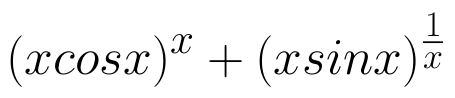. (View Answer Video)

Question 2 : Differentiate the function w.r.t.x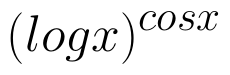. (View Answer Video)

Question 3 : Findfor the function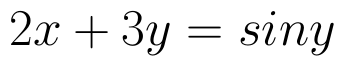. (View Answer Video)

Question 4 :  Find the second order derivative of the function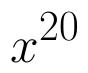. (View Answer Video)

Question 5 : Differentiate w.r.t.x the function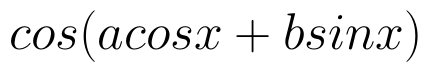, for some constant a and b. (View Answer Video)